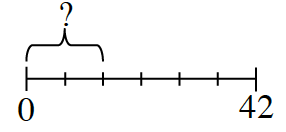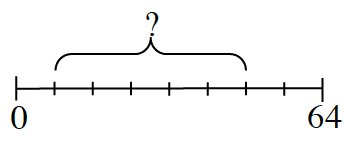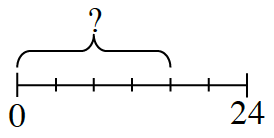Home > CC2MN > Chapter cc24 > Lesson cc24.1.1 > Problem4-9

4-9.

Find the missing parts of each number line. Assume that the lines have been split into equal parts. Homework Help ✎

1.Find the length of each segment. Then multiply that number by the number of segments in the missing part.

The segments are $7$ units long, and there are two segments in the missing part.

$14$

1.Use the method from part (a).

1.Use the method from part (a).